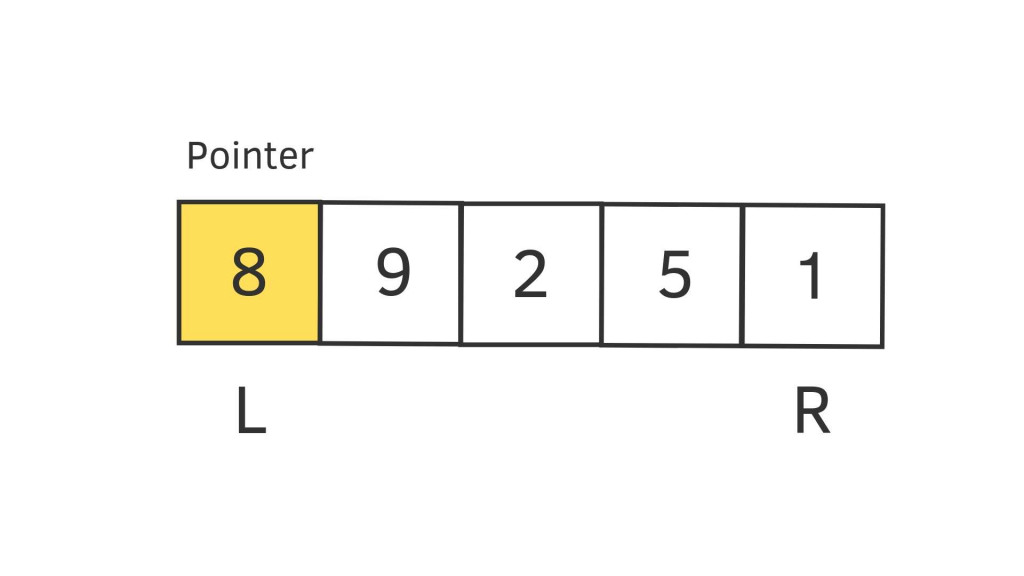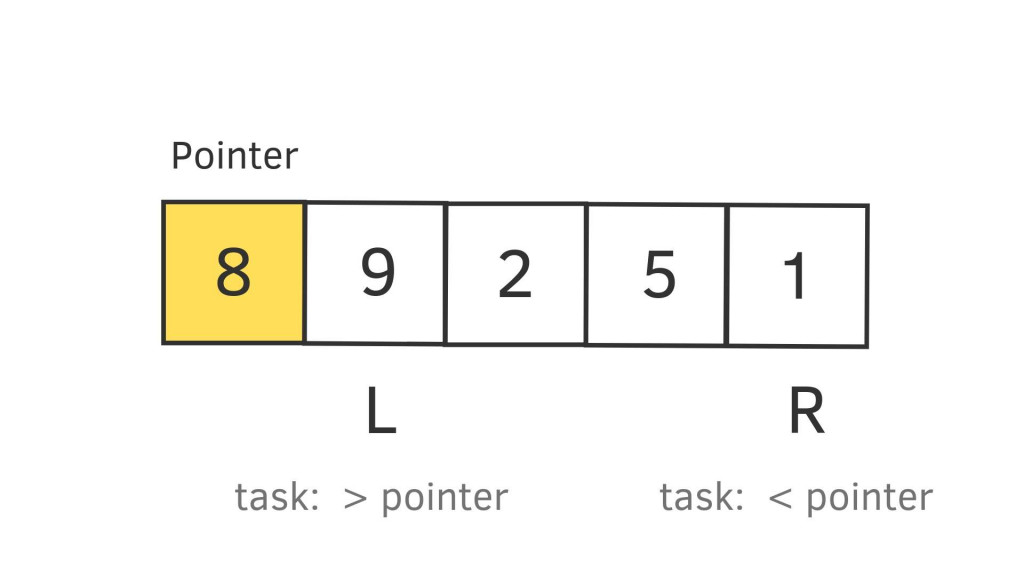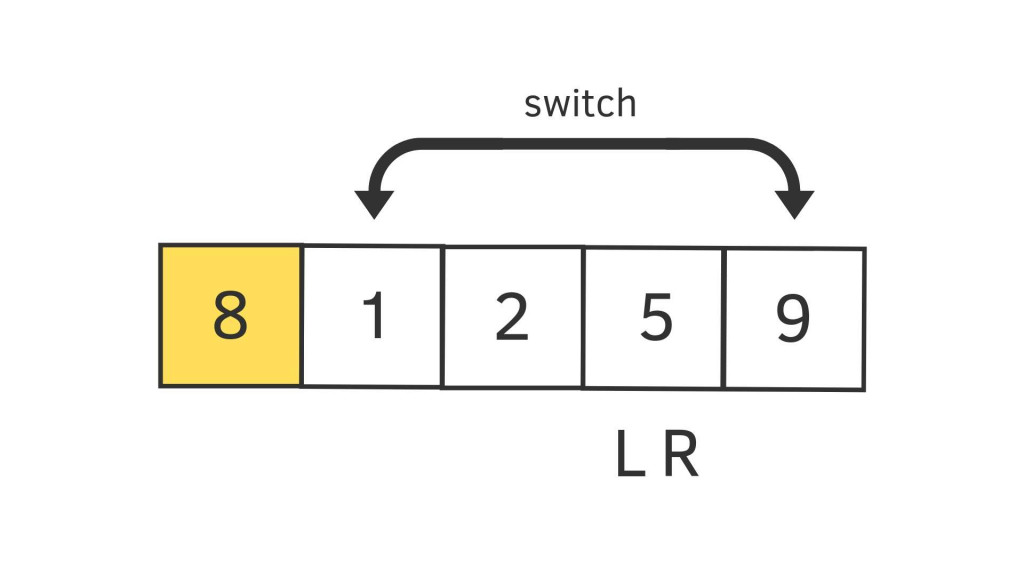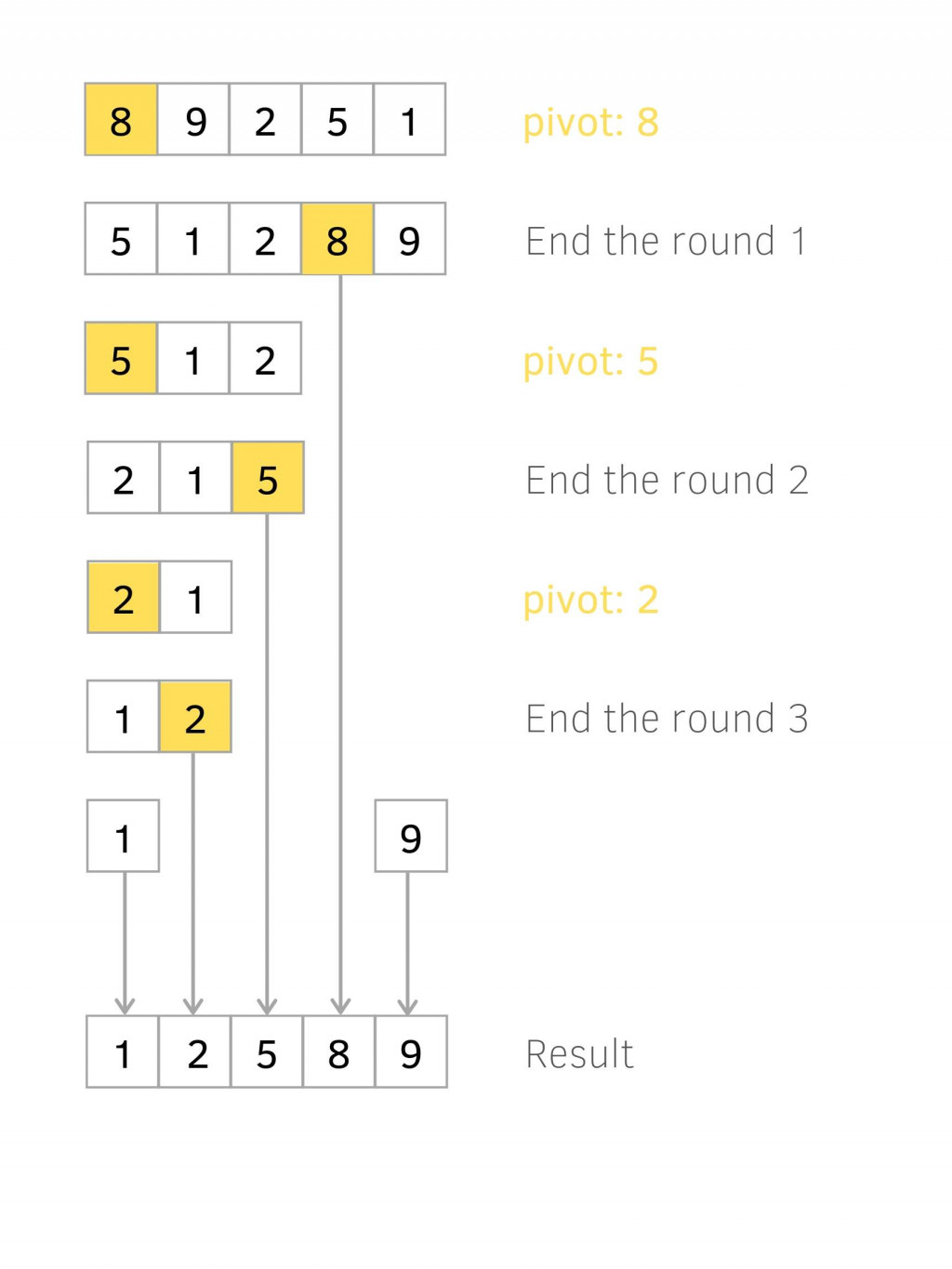#DAY 22
2
Software Development

# Big O(n logn)：Quick Sort 快速排序

Quick Sort 會先選一個基準數 (pivot)，然後其他元素跟 pivot 輪流比較，小的放前大的放後。pivot 兩邊也會切割成更小的 Array 直到只剩一個值，最後再組裝。### 觀察步驟

``````// before sort
[8, 9, 2, 5, 1]
// after sort
[1, 2, 5, 8, 9]
``````• 隨便選一個值當基準數 (pivot)
• 最左邊會有一個 pointer (指標) L，最右邊位置也會有一個 pointer R
• R 往左移動直到找到一個小於 pivot 停下來
• L 往右移動直到找到一個大於 pivot 停下來• 值交換位置，L 跟 R 繼續移動• 若一直沒有停下來，那 L 跟 R 重疊時，重疊的值跟基準(pivot)交換，當回合結束。

#### 切割

• 以基準值出發分為左右兩塊 Array
• 繼續重覆上述步驟一直到 Array 只剩一個

### 實際操作

``````// 第一輪
// 第一次交換
[8, 9, 2, 5, 1]  // Determine pivot, I choose 8
-

[8, 9, 2, 5, 1] // start pointer at left and right
-
L           R

[8, 9, 2, 5, 1] // R 找到一個 < 8 的停下來，因為 1 < 8 所以第一步就停了
-
L           R

[8, 9, 2, 5, 1] // L 找到一個 > 8 的停下來，他往右移到 9 就停住
-
L        R

[8, 1, 2, 5, 9] // When L & R all stop, swich position
-
L        R

// 第二次交換

[8, 1, 2, 5, 9] // 5 < 8, so stop
-
L     R

[8, 1, 2, 5, 9] // L 碰到 R 了但都沒發現 > 8 的，但碰到代表第一輪結束，把 pivot 跟 5 交換
-
LR

[5, 1, 2, 8, 9]
-        -
``````

``````// 第二輪
[5, 1, 2]  // Determine pivot
-

[5, 1, 2]  // start pointer at left and right
-
L     R

[5, 1, 2]  // R < 5, so stop
-
L     R

[5, 1, 2]  // L 沒找到 > 5 的直到碰到 R
-
LR

[2, 1, 5]  // 碰到代表這回合結束，交換位置
-     -

// 第三輪
[2, 1]  // Determine pivot
-

[2, 1]  // start pointer at left and right
-
L  R

[2, 1]  // 1 < 2, so stop
-
L  R

[2, 1]  //  碰到
-
LR

[1, 2]  //  碰到所以交換

// 第四輪   就不用排了
``````### 程式碼

``````let data = [8, 9, 2, 5, 1];

let quickSort = (arr, L, R) => {
// 實作劃分過程
const partition = (array, L, R) => {
let pivot = L + 1;
for (let i = L + 1; i <= R; i++) {
if (array[i] < array[L]) {
swap(array, i, pivot);
pivot++;
}
}

// 記得把 pivot 跟最後一個比它小的元素互換
swap(array, L, pivot - 1);
return pivot - 1;
}
const _quickSort = (array, L, R) => {
if (L >= R) return array;

// 在 partition 裡面調整數列，並且回傳 pivot 的 index
const pivotIndex = partition(array, L, R);
_quickSort(array, L, pivotIndex - 1);
_quickSort(array, pivotIndex + 1, R);
return array;
};
return _quickSort(arr, 0, arr.length - 1);
}

// array 裡面的值自己交換 array[index1] = array[index2]
function swap(arr, index1, index2) {
// es6 Destructuring
[arr[index1], arr[index2]] = [arr[index2], arr[index1]];
}

console.log(quickSort(data, 0, data.length - 1))
``````

``````function quickSort(arr) {
if (arr.length < 2) return arr
const [p, ...ary] = arr
const left = [], right = []

ary.forEach(c => {
if (c < p) left.push(c)
else right.push(c)
})

return [...quickSort(left), p, ...quickSort(right)]
}
``````

``````如有錯誤或需要改進的地方，拜託跟我說。

``````

### 1 則留言

1

`swap` 裡面得 `tmpValue` 應該要用 `const`

hannahpun iT邦新手 5 級 ‧ 2019-09-24 00:05:52 檢舉